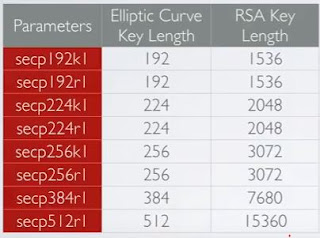# New Technology

Trending Technology Machine Learning, Artificial Intelligent, Block Chain, IoT, DevOps, Data Science

## Search

Elliptic Curve key Pair Generation
• Blockchain implementations such as Bitcoin or Ethereum uses Elliptic Curves (EC) to generate private and public key pairs.
• Elliptic Curve Cryptography (ECC) was invented by Neal Koblitz and Victor Miller in 1985.
• A 256-bit ECC public key provides comparable security to a 3072-bit RSA public key. The primary advantage of using Elliptic Curve based cryptography is reduced key size and hence speed.
• Elliptic curves have nothing to do with ellipse.Ellipses are formed by quadratic curves (x2). Elliptic curves are always cubic (x3).
Standards for Efficient Cryptography Group
• The Standards for Efficient Cryptography Group (SECG) is an international consortium to develop commercial standards for efficient and interoperable cryptography based elliptic curve cryptography (ECC).
• The SECG website is http://www.secg.org
• The SECG has published a document with a recommended set of elliptic curve domain parameters, referred by the letters p, a, b, G, n, h. The data set { p, a, b, G, n, h} is collectively referred to as the Elliptic Curve Domain Parameters.
• The parameters have been give nicknames to enable them to be easily identified. For example: secp256klElliptic Curve Domain Parameters

• In this table you will find a set of elliptic curve domain parameters.
• The elliptic curves uses smaller key sizes with respect to RSA providing comparable security.

SECP256K1

SECP256K1: Parameter P
• A finite field is a field with a finite number of element, defined by parameter p, which is a prime number. Thus the finite field Fp = {0,....p-1}
• This means that modulo p should be used in the equation:
➝ The EC equation: y2 = x3 + ax + b
➝ The EC equation with modulo operation: y2 = x3 + ax + b (mod p)

SECP256K1 : Parameter G
• The basepoint G, also known as the generator or primitive element, is a predetermined point (XG, YG) on the elliptic curve that everyone uses to compute other points on the curve.
SECP256K1 : Parameter N
• In my discrete logarithm I have explained what a cyclic group is. When you apply a certain number of operation to base point G, the cycle starts all over again in the same order.When the next cycle starts the first time it is indicated by parameter n which is called the order of base point G.
• n= FFFFFFFF FFFFFFFF FFFFFFFF FFFFFFFF BAAEDCE6 AF48A03B BFD25E8C D0364141
• The parameter n determines what the maximum value is that can be turned into a private key. Any 256-bit number in the range [1, n-1] is a valid private key.
SECP256K1 : Parameter H
• The parameter h is called the cofactor and has the constant value 1.
• Because it has value 1 it does not play a role in the key generation and 1 therefore will not elaborate on the purpose of this parameter.
Dot Operations
• There are two operations often called dot operations which can be applied to a base point (aka generator G) (XG, YG) on the elliptic curve:
- Point doubling
• The elliptic curve (y2 = x3 + 7) has the following properties:
- If a line intersects two points P and Q, it intersects a third point on the curve-R.
- If a line is tangent to the curve, it intersects another point on the curve.
- All vertical lines intersects the curve at infinity.

• Adding two points P and Q on a elliptic curve (P≠Q).
• Geometry approach:
- Draw a straight line between P(x1, y1) and Q(x2,y2).
- The line will intersect the elliptic curve at exactly one more point -R.
- The reflection of the point-R with respect to x-axis gives the point R(x3,y3), which is the results of addition of points P and Q.

Point Doubling
• Point doubling of point P on an elliptic curve. It is the same as moving point Q to same location as point P (P = Q)
• Geometry approach:
- Draw a tangent line to the elliptic curve at point P.
- The line intersect the elliptic curve at the point-R.
- The reflection of the point-R with respect to x-axis gives the point R, which is the results of doubling of point P.
• Point doubling does not mean multiplying the x or y coordinates of P. It is just name give for this approach.

Mathematical Equations

1.